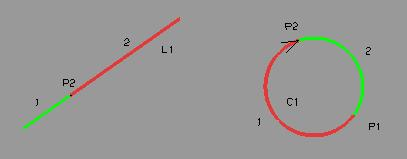```<dy 090920 ok>

CUT, Trim
Intersect, intersection curves (INT)
Transform (move, rotate) (TRA)
Project
Mirror

CUT (trim) obj          CUT
Trim / limit linear elements (lines, curves).
(Separate with one or two cutting elements).

Caution: currently only for curves, not yet for surfaces/solids and CCVs.

CUT Obj CuttingElement (CuttingElement2) (Variant) (REVerse)

Obj: this element will be changed (trimmed).
If it is already trimmed, also its parent-object will be displayed
in the selection-list.

Cutting element: the object will be separated with this element
one (optionally two) elements, e.g. points.
For curves, parameter values (0-1) can be used.
Two cutting elements: result is the mid-part.

Variant: which of the resulting elements will be retained
Change with the Page Up/Page Down keys
Default or “1” saves the first resulting object

REVerse: Reverse the direction of the resulting curve.Example:
L20 = P(-60 30 0) P(20 70 0)
C20 = P(-14 40 0) VAL(32) DZ
S20 = CUT C20 L20 L20
# Circle C20 is limited at both ends (trimmed).

# Parameter values as cutting element:
S21 = CUT S20 0.33 0.66

INT (intersect) obj     INT
Create intersection points / intersection curves.

resulting_object = INT Obj1 Obj2 [version] [POL]

Obj1: object 1
Curve or surface. Continue with Tab-key

Obj1: object 2
Curve or surface
For curves, parameter values (0-1) can be used.

Version: Define which element of the result is to be saved.
Select using the PageUp/PageDown keys.
Default or "1": Saves the first result object (point or curve)
"2" would select the second one ..

POL: Only for curves as a result
output is polygon or spline (default is spline)

Example:
P20 = P(-62.5 -63.7 0)
S20 = CCV P20 P(-20 -70) P(-40 -15) P20
A20 = S20
#
P21 = P(-60 -110 20)
P22 = P(S20 MOD (2) 1)
S21 = POL P21 P20 P22 P21
A21 = S21
#
R20 = X(-35)
#
# A group of Areas:
S22 = INT U(A20 A21) R20 POL

# point from intersection line x surface:
P1=INT L1 A1

# curve from intersection curve X surface:
# (curve: circle, ellipsis, B-Spline) not yet implemented

# curve from intersection surface x surface:
S1=INT A1 A2

P4=INT C1 C2 MOD(2)   # intersection-point nr. 2 from intersection C1 - C2

TRAnslate obj (VC)      TRA
Translate an object

Result = Object TRA Obj_to_move vector [length]
TRA Obj_to_move: this object is moved
Vector
length       vectorlength (optional)
replace-duplicat   if parentobject should be kept or hidden; default = keep.
press Page-down to keep ("REPL").

A new, translated object (a copy) will be created.
Permanent translation of the selected object: use "Group/move objects of group"

# Objects to move a vector (REV: opposite direction).
# Obj = vec TRA obj/traVC [REV]
P20 = P(100,0,0)
P21 = TRA P20 D(0 0 100)

# "TRafo transl"  (define Transformation)
T20 = D(10 10 10)
# "TRAnslate obj (VC)"  and keep parent
P22 = TRA P21 T20
# TraVC (T20 in the example): see Transformation
# replace parent
P23=TRA P22 D(0 0 100) REPL

TRAnslate obj (PLN)     TRA
Move an object to a different reference system.
Each layer can be used as a reference system.

Result object = TRA object reference system [REV]
Reference System
REV: Move an object from a reference system back into the main location.

Example:
R20 = P(100 100 25) D (-1 0 2)
L20 = P(100 100 25) P (100 120 25)
# Move line L20 to the Refsys R20
L21 = TRA L20 R20
# Move a line back into the main location.
L22 = TRA L21 R20 REV

TRAnsform obj (Tra)     TRA
Transformation of objects (move, rotate).
The transformation must be defined previously!

Result object = TRA object transformation
Transformation: Select example using the PageUp/PageDown keys

Translation:
P20 = P(100 0 0)
Translation # Z in the direction of 100 mm
T20 = DZ VAL(100)
P21 = TRA P20 T20

Rotation:
P20 = P(100 0 0)
# Define a 30-Grad-Rotation to line L20
L20 = P(50 0 0) P(50 0 10)
T20 = ROT L20 ANG(90)
# P20 rotate
P21 = TRA P20 T20

reverse curve      REV
Reverse direction of curve.
The resulting curve looks identical.

Project obj        PRJ
Project points or linear elements (lines, circles, curves) onto
an other linear element or on a surface.

Resulting_object = PRJ object targetObject [direction] [solutionNr]

object:          project this object -
targetObject:    - onto this object.
direction:       projection-direction, a vector; none: normal to targetObject.
solutionNr:      1-n, PgUp/PgDown keys.

Example:
P21=PRJ P20 R20
# Point P20 will be projected on the plane R20; direction is perpendicular.

S20 = PRJ S20 R20 D20
# Curve S20 will be projected in direction of D20 on the plane R20

Parallel obj (offset)       PARL
Create a parallel object.
Until now only for planar curves.
basic obj                 select curve
offset dist               keyIn distance
[basic plane/surf]        optional - define plane of curve
[nr of contour; 0=all]    if result has more than one curves:
no input or 1 gives first curve ..
[rounded/edges]           no rounding of corners; until now only for circle, line

Example:
S23=PARL S21 VAL(3)
# creates curve S21 parallel to curve S20, distance is 3.

Reflect objects across a line
Currently, only for linear elements, plane, not surfaces.

resulting object = MIR object line

Example:
S20=MIR S20 L20
# Curve S20 is mirrored across line L20

Mirror obj (Plane)      MIR
Reflect objects across a plane
Currently, only for linear elements, not surfaces

Result object = MIR object plane

Example:
S20 = MIR S20 R20
# Curve S20 is mirrored across plane R20

ISOparametric Curve from Surf         ISO
Extracts an isoparametric curve from a surface.
Currently only B-Spline surface. Entering point or parameter value has not yet been implemented

Input:
Surface
Parameter value (0-1)
Default: lengthwise Direction; "CX" defines lateral direction.
(Modifier CX = across; Default = along)

S = parameter surf ISO/point (direction U/V)

Example:
# Cross-section curve in the middle of the surface A20:
S20 = ISO A20 .5 CX

```